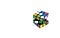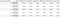# Tips on Principal Component Analysis

## How to select the number of principal components and application of PCA to new observationsPhoto by Volodymyr Hryshchenko on Unsplash

# Introduction

Principal Component Analysis (PCA) is an unsupervised technique for dimensionality reduction.

What is dimensionality reduction?

Let us start with an example. In a tabular data set, each column would represent a feature, or dimension. It is commonly known that it is difficult to manipulate a tabular data set that has a lot of columns/features, especially if there are more columns than observations.

Given a linearly modelable problem having a number of features p=40, then the best subset approach would fit a about trillion (2^p-1) possible models and submodels, making their computation extremely onerous.

How does PCA come to aid?

PCA can extract information from a high-dimensional space (i.e., a tabular data set with many columns) by projecting it onto a lower-dimensional subspace. The idea is that the projection space will have dimensions, named principal components, that will explain the majority of the variation of the original data set.

How does PCA work exactly?

PCA is the eigenvalue decomposition of the covariance matrix obtained after centering the features, to find the directions of maximum variation. The eigenvalues represent the variance explained by each principal component.

The purpose of PCA is to obtain an easier and faster way to both manipulate data set (reducing its dimensions) and retain most of the original information through the explained variance.

The question now is

How many components should I use for dimensionality reduction? What is the “right” number?

In this post, we will discuss some tips for selecting the optimal number of principal components by providing practical examples in Python, by:

1. Observing the cumulative ratio of explained variance.
2. Observing the eigenvalues of the covariance matrix
3. Tuning the number of components as hyper-parameter in a cross-validation framework where PCA is applied in a Machine Learning pipeline.

Finally, we will also apply dimensionality reduction on a new observation, in the scenario where PCA was already applied to a data set, and we would like to project the new observation on the previously obtained subspace.

# Environment set-up

At first, we import the modules we will be using, and load the “Breast Cancer Data Set”: it contains 569 observations and 30 features for relevant clinical information — such as radius, texture, perimeter, area, etc. — computed from digitized image of aspirates of breast masses, and it presents a binary classification problem, as the labes are only 0 or 1 (benign vs malignant), indicating whether a patient has breast cancer or not.

The data set is already available in scikit-learn:

Without diving deep into the pre-processing task, it is important to mention that the PCA is affected by different scales in the data.

Therefore, before applying PCA the data must be scaled (i.e., converted to have mean=0 and variance=1). This can be easily achieved with the scikit-learn StandardScaler object:

This returns:

`Mean:  -6.118909323768877e-16Standard Deviation:  1.0`

Once the features are scaled, applying the PCA is straightforward. In fact, scikit-learn handles almost everything by itself: the user only has to declare the number of components and then fit.

Notably, the scikit-learn user can either declare the number of components to be used, or the ratio of explained variance to be reached:

• pca = PCA(n_components=5): performs PCA using 5 components.
• pca = PCA(n_components=.95): performs PCA using a number of components sufficient to consider 95% of variance.

Indeed, this is a way to select the number of components: asking scikit-learn to reach a certain amount of explained variance, such as 95%. But maybe we could have used a significantly lower amount of dimensions and reach a similar variance, for example 92%.

So, how do we select the number of components?

# 1. Observing the ratio of explained variance

PCA achieves dimensionality reduction by projecting the observations on a smaller subspace, but we also want to keep as much information as possible in terms of variance.

So, one heuristic yet effective approach is to see how much variance is explained by adding the principal components one by one, and afterwards select the number of dimensions that meet our expectations.

It is very easy to follow this approach thanks to scikit-learn, that provides the explained_variance_ratio_ property to the (fitted) PCA object:

From the plot, we can see that the first 6 components are sufficient to retain the 89% of the original variance.

This is a good result, if we think that we started with a data set of 30 features, and that we could limit further analysis to only 6 dimensions without loosing too much information.

# 2. Using the covariance matrix

Covariance is a measure of the “spread” of a set of observations around their mean value. When we apply PCA, what happens behind the curtain is that we apply a rotation to the covariance matrix of our data, in order to achieve a diagonal covariance matrix. In this way, we obtain data whose dimensions are uncorrelated.

The diagonal covariance matrix obtained after transformation is the eigenvalue matrix, where the eigenvalues correspond to the variance explained by each component.

Therefore, another approach to the selection of the ideal number of components is to look for an “elbow” in the plot of the eigenvalues.

Let us observe the first elements of the covariance matrix of the principal components. As said, we expect it to be diagonal:

Indeed, at first glance the covariance matrix appears to be diagonal. In order to be sure that the matrix is diagonal, we can verify that all the values outside of the main diagonal are almost equal to zero (up to a certain decimal, as they will not be exactly zero).

We can use the assert_almost_equal statement, that leads to an exception in case its inner condition is not met, while it leads to no visible output in case the condition is met. In this case, no exception is raised (up to the tenth decimal):

The matrix is diagonal. Now we can proceed to plot the eigenvalues from the covariance matrix and look for an elbow in the plot.

We use the diag method to extract the eigenvalues from the covariance matrix:

We may see an “elbow” around the sixth component, where the slope seems to change significantly.

Actually, all these steps were not needed: scikit-learn provides, among the others, the explained_variance_ attribute, defined in the documentation as “The amount of variance explained by each of the selected components. Equal to n_components largest eigenvalues of the covariance matrix of X.”:

In fact, we notice the same result as from the calculation of the covariance matrix and the eigenvalues.

# 3. Applying a cross-validation procedure

Although PCA is an unsupervised technique, it might be used together with other techniques in a broader pipeline for a supervised problem.

For instance, we might have a classification (or regression) problem in a large data set, and we might apply PCA before our classification (or regression) model in order to reduce the dimensionality of the input dataset.

In this scenario, we would tune the number of principal components as a hyper-parameter within a cross-validation procedure.

This can be achieved by using two scikit-learn object:

• Pipeline: allows the definition of a pipeline of sequential steps in order to cross-validate them together.
• GridSearchCV: performs a grid search in a cross-validation framework for hyper-parameter tuning (= finding the optimal parameters of the steps in the pipeline).

The process is as follows:

1. The steps (dimensionality reduction, classification) are chained in a pipeline.
2. The parameters to search are defined.
3. The grid search procedure is executed.

In our example, we are facing a binary classification problem. Therefore, we apply PCA followed by logistic regression in a pipeline:

This returns:

`Best parameters obtained from Grid Search:{'log_reg__C': 1.2589254117941673, 'pca__n_components': 9}`

The grid search finds the best number of components for the PCA during the cross-validation procedure.

For our problem and tested parameters range, the best number of components is 9.

The grid search provides more detailed results in the cv_results_ attribute, that can be stored as a pandas dataframe and inspected:Some of the columns in the dataframe obtained by storing the cv_results_ attribute output.

As we can see, it contains detailed information on the cross-validated procedure with the grid search.

But we might be not interested in seeing all the iterations performed by the grid search. Therefore, we can get the best validation score (averaged on all folds) for each number of components, and finally plot them together with the cumulative ratio of explained variance:

From the plot, we can notice that 6 components are enough to create a model whose validation accuracy reaches 97%, where considering all 30 components would lead to a 98% validation accuracy.

In a scenario with a significant number of features in a input data set, reducing the number of input features with PCA could lead to significant advantages in terms of:

1. Reduced training and prediction time.
2. Increased scalability.
3. Reduced training computational effort.

While, at the same time, by choosing the optimal number of principal components in a pipeline for a supervised problem, tuning the hyper-parameter in a cross-validated procedure, we would ensure to retain optimal performances.

Although, it must be taken into account that in a data set with many features the PCA itself may prove computationally expensive.

# How to apply PCA to a new observation?

Now, let us suppose that we have applied the PCA to an existing data set and kept (for example) 6 components.

At some point, a new observation is added to the data set and needs to be projected on the reduced subspace obtained by PCA.

How can this be achieved?

We can perform this calculation manually through the projection matrix.

Therefore, we also estimate the error in the manual calculation by checking if we would get the same output as “fit_transform” on the original data:

The projection matrix is orthogonal, and the manual reduction provides a fairly reasonable error.

We can finally obtain the projection by the multiplication between the new observation (scaled) and the transposed projection matrix:

This returns:

`[-3.22877012 -1.17207348  0.26466433 -1.00294458  0.89446764  0.62922496]`

That’s it! The new observation is projected to the 6-dimensional subspace obtained with PCA.

# Conclusion

This tutorial is meant to provide a few tips on the selection of the number of components to be used for the dimensionality reduction in the PCA, showing practical demonstrations in Python.

Finally, it is also explained how to perform the projection onto the reduced subspace of a new sample, information which is rarely found on tutorials on the subject.

This is but a brief overview. The topic is far broader and it has been deeply investigated in literature.

# References

1. Jake VanderPlas, “Python Data Science Handbook”, O’Reilly, 2016.
2. The scikit-learn documentation, in particular this example on PCA, that inspired the cross-validation section.

Thanks to Linda Chen

## More from Nicolo Cosimo Albanese

Data Scientist & philomath.